Note: The other languages of the website are Google-translated. Back to English

## 如何在Excel中將IF函數與AND，OR和NOT一起使用？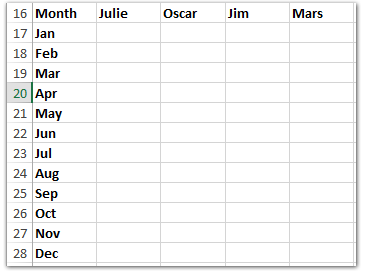#### 如何在Excel中使用IF函數

Excel中IF函數的基本形式如下所示： = IF（邏輯測試，value_if為true，value_if_false）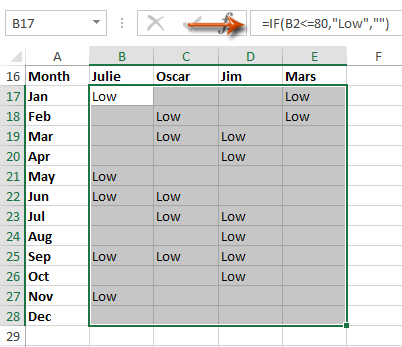#### 輕鬆地將多個工作表/工作簿/ CSV文件組合到一個工作表/工作簿中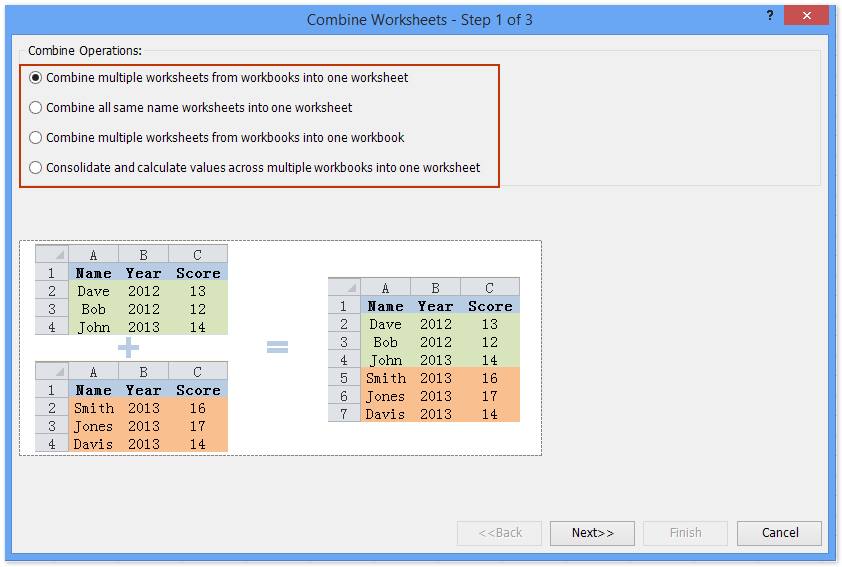#### 如何在Excel中使用If函數和AND函數

Excel中AND函數的基本形式是 = AND（邏輯1，邏輯2，邏輯3，…等）#### 如何在Excel中使用IF函數和OR函數

Excel中OR功能的基本形式是 = OR（邏輯1，邏輯2，邏輯3，等等）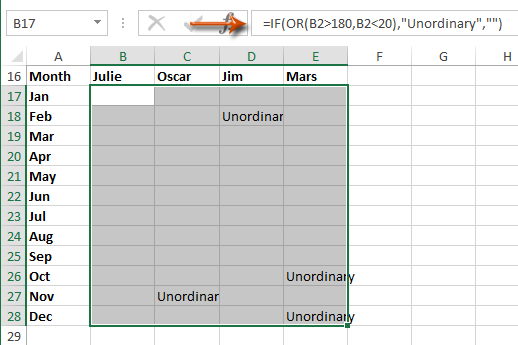#### 如何在Excel中將多個IF函數合併為一個公式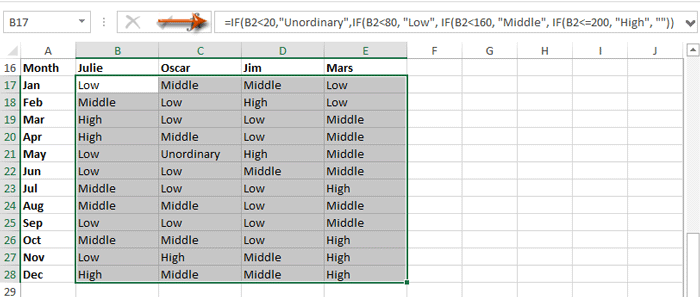公式太難記了嗎？ 將公式另存為“自動文本”條目，以供日後再次使用！ 閱讀全文...     免費試用

### 最佳辦公效率工具

#### Kutools for Excel 解決了你的大部分問題，並將你的生產力提高了 80%

• 重用: 快速插入 複雜的公式，圖表 以及您以前使用過的任何東西； 加密單元 帶密碼 創建郵件列表 並發送電子郵件...
• 超級公式欄 （輕鬆編輯多行文本和公式）； 閱讀版式 （輕鬆讀取和編輯大量單元格）； 粘貼到過濾範圍...
• 合併單元格/行/列 不會丟失數據； 拆分單元格內容； 合併重複的行/列...防止細胞重複； 比較範圍...
• 選擇重複或唯一 行; 選擇空白行 （所有單元格都是空的）； 超級查找和模糊查找 在許多工作簿中； 隨機選擇...
• 確切的副本 多個單元格，無需更改公式參考； 自動創建參考 到多張紙； 插入項目符號，複選框等...
• 提取文字，添加文本，按位置刪除， 刪除空間; 創建和打印分頁小計； 在單元格內容和註釋之間轉換...
• 超級濾鏡 （將過濾方案保存並應用於其他工作表）； 高級排序 按月/週/日，頻率及更多； 特殊過濾器 用粗體，斜體...
• 結合工作簿和工作表; 根據關鍵列合併表； 將數據分割成多個工作表; 批量轉換xls，xlsx和PDF...
• 超過 300 項強大的功能. 支持 Office / Excel 2007-2021 和 365。支持所有語言。 在您的企業或組織中輕鬆部署。 完整功能 30 天免費試用。 60 天退款保證。#### Office選項卡為Office帶來了選項卡式界面，使您的工作更加輕鬆

• 在Word，Excel，PowerPoint中啟用選項卡式編輯和閱讀，發布者，Access，Visio和Project。
• 在同一窗口的新選項卡中而不是在新窗口中打開並創建多個文檔。
• 將您的工作效率提高 50%，每天為您減少數百次鼠標點擊！how do i make this formulas talk to one another: =IF(OR(AND(MID(K2,6,1)="N",(MID(K2,6,1)="C"),(MID(K2,6,1)="H"),(MID(K2,6,1)="I"),(MID(K2,6,1)="B"),(MID(K2,6,1)="F"),(MID(K2,6,1)="L"),(MID(K2,6,1)="M"),(MID(K2,6,1)="P"),(MID(K2,6,1)="R"),(MID(K2,6,1)="P"),ISTEXT(G2)="61"),OR(AND(RIGHT(K2,2)=G2))),"Good","Review") =IF(AND(RIGHT(K2,2)=G2),"Good","Review") or =IF(NOT(OR(AND(MID(K2,6,1)="N",(MID(K2,6,1)="C"),(MID(K2,6,1)="H"),(MID(K2,6,1)="I"),(MID(K2,6,1)="B"),(MID(K2,6,1)="F"),(MID(K2,6,1)="L"),(MID(K2,6,1)="M"),(MID(K2,6,1)="P"),(MID(K2,6,1)="R"),(MID(K2,6,1)="P"),ISTEXT(G2)="61"),(RIGHT(K2,2)=G2))),"Good","Review")

“我有收費計算，例如收費金額為 0，然後下一列應為 0。

0 0
45 250
250 250
255 255
300 300
0 0
1 錯誤

",0)))))))

Try this: =IF(OR(H16=0,H16=""),"A",IF(H16=8,"R",IF(H16<7.9,"L","OT")))

=IF(OR((I2=(T2+U2),(I2=(U2+V2)),"Closed","OPEN")

=IF(OR(I2=T2+U2,I2=U2+V2),"CLOSED","OPEN")

=IF(AND(B2="Prudential",OR(C2={"10 年期限","15 年期限","20 年期限","30 年期限"})),D2*0.75,(IF( C2="UL",D2*0.8,"0")))

5 = 100％
4= 99.9% 至 90%
3= 90% 至 85%
2= 85% 至 80%
1= <79.99

OR A1 = "TOPS" AND A9 <5，則為 5，否則為 A9

``=ROUND((IFERROR((IF(OR(AND(\$I9="BOTTOMS",BL9>=\$BY\$3),AND(\$I9="TOPS",BL9>=\$BY\$4)),BL9,VLOOKUP(\$I9,\$BX\$3:\$BY\$4,2,FALSE))),0)),0)``

I9 - 根據輸入的商品代碼填充“BOTTOMS”或“TOPS”
BL9 - 商店指數定義的最小集合
BY9 - 買方定義的最小設置（即底部的 14 個單位和頂部的 5 個單位）
BX\$3:\$BY\$4 - 如果 if 語句為假，則查找買方定義的最小集合

1. 這個數字是超過 1000 英鎊還是低於 -1000 英鎊

2. Plus 是下一個單元格超過 55% 或更多

=IF(AND(D26="",E26=""),(C26-L7),IF(AND(D26<>"",E26=""),D26-L7,E26-L7))

III
II
II
II

II
II
II
II

C。 如果工作人員不符合這兩個標準，該函數應返回文本 No。

https://support.office.com/en-us/article/IF-function-69aed7c9-4e8a-4755-a9bc-aa8bbff73be2

A 列有數值。 C & D 列有文字。

A1:A2 = 1

A3:A4 = 2

C1=狗
C2=貓
C3=狗
C4：馬

D1=貓
D2=狗
D3=馬
D4=狗

https://support.office.com/en-us/article/IF-function-69aed7c9-4e8a-4755-a9bc-aa8bbff73be2

=IF(A3>0,"Welcome",IF(B3>0,"Win",IF(C3>0,"Will","HI")))

0   字符

## 關注我們

Microsoft和Office徽標是Microsoft Corporation在美國和/或其他國家的商標或註冊商標。